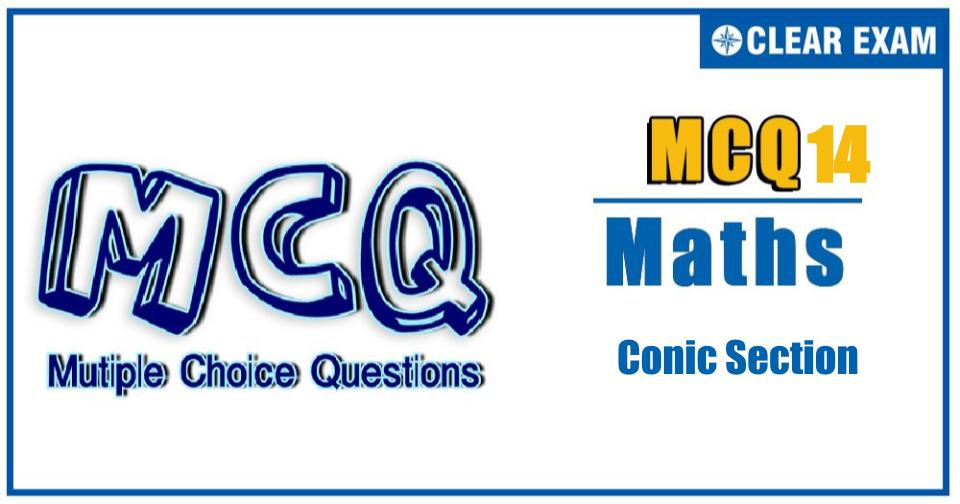[LATEST]\$type=sticky\$show=home\$rm=0\$va=0\$count=4\$va=0

In JEE exams, Conic section is one of the most important topic of Mathematics that comes under Coordinate Geometry, it has total of 15 percent weightage out of which 6% questions are asked in JEE mains and 9% in Advance.

Q1. The exhaustive set of values of α^2 such that there exists a tangent to the ellipse x22 y22 such that the portion of the tangent intercepted by the hyperbola α2 x2-y2=1 subtends a right angle at the centre of the curves is
•  [(√5+1)/2, 2]
•  (1, 2]
•  [(√5-1)/2, 1)
•  [(√5-1)/2, 1) ∪ (1,(√5+1)/2]
Q2. If P(x1,y1 ),Q(x2,y2 ),R(x3,y3 ) and S(x4,y4) are four con-cyclic points on the rectangular hyperbola xy=c22, then coordinates of the orthocentre of the triangle PQR is
•  (x4,-y4)
•  (x_4,y_4)
•  (-x4,-y4)
•  (-x4,y4)
Q3. Angle subtended by common tangents of two ellipses 4(x-4)2+25y2=100 and 4(x+1)2=4 at origin is
•  π/3
•  π/4
•  π/6
•  π/2

Q4.   From a point (sin⁡θ,cos⁡θ) if three normals can be drawn to the parabola y2=4ax then the value of ‘a’ is
•  (1/2,1)
•  (1/2 0)
•  [1/2,1]
•  (-1/2,0)∪(0,1/2)
Q5. The tangent at a point P on the hyperbola x2/a2 -y2/b2 =1 meets one of the directrix in F. If PF subtends an angle θ at the corresponding focus, then θ equals
•  π/4
•  π/2
•  3π/4
•  π
Q6. Let y=f(x) be a parabola, having its axis parallel to y–axis, which is touched by the line y=x at x=1, then
•  2f(0)=1-f'(0)
•  f(0)+f'(0)+f''(0)=1
•  f'(1)=1
•  f'(0)=f'(1)
Q7. The curve described parametrically by x=t2+ t+1,y=t2-t+1 represents
•  A pair of straight lines
•  An ellipse
•  A parabola
•  A hyperbola
Q8. Locus of the feet of the perpendiculars drawn from either focus on a variable tangent to the hyperbola 16y2-9x2=1 is
•  x2+y2=9
•  x2+y2=1/9
•  x2+y2=7/144
•   x2+y2=1/16
Q9.A line of fixed length a+b moves so that its ends are alwayes on two fixed perpendicular straight lines, then the locus of a point, which divides this line into portions of lenghts a and b is a/an
•  Ellipse
•  Parabola
•  Straight line
•  None of these
Q10.A variable chord of the hyperbola x2/a2 -y2/b2 =1 (b>a) subtends a right angle at the centre of the hyperbola, if this chord touches
•  A fixed circle concentric with the hyperbola
•  A fixed ellipse concentric with the hyperbola
•  A fixed hyperbola concentric with the hyperbola
•  A fixed parabola having vertex at (0, 0)Written by: AUTHORNAME

AUTHORDESCRIPTIONWant to know more

Please fill in the details below:

Latest NEET Articles\$type=three\$c=3\$author=hide\$comment=hide\$rm=hide\$date=hide\$snippet=hide

Name

ltr
item
BEST NEET COACHING CENTER | BEST IIT JEE COACHING INSTITUTE | BEST NEET, IIT JEE COACHING INSTITUTE: Conic Section Quiz-14
Conic Section Quiz-14
https://1.bp.blogspot.com/-kLXRkC7dCjA/YPPass7p3rI/AAAAAAAAYPk/uC3DtVhHghIJwd75M8HWzFGaSX6DITFmwCLcBGAsYHQ/s0/14.jpg
https://1.bp.blogspot.com/-kLXRkC7dCjA/YPPass7p3rI/AAAAAAAAYPk/uC3DtVhHghIJwd75M8HWzFGaSX6DITFmwCLcBGAsYHQ/s72-c/14.jpg
BEST NEET COACHING CENTER | BEST IIT JEE COACHING INSTITUTE | BEST NEET, IIT JEE COACHING INSTITUTE
https://www.cleariitmedical.com/2021/07/conic-section-quiz-14.html
https://www.cleariitmedical.com/
https://www.cleariitmedical.com/
https://www.cleariitmedical.com/2021/07/conic-section-quiz-14.html
true
7783647550433378923
UTF-8